# 前言：

∇ \nabla 联系方式：

e-mail: FesianXu@gmail.com

QQ: 973926198

github: https://github.com/FesianXu# 1，在分类问题中，损失函数及其快速得下降为0.0000

L c l s = − ∑ i = 1 n y i log ⁡ y ^ i = − y T log ⁡ y ^ y ∈ R n , y ^ ∈ R n (1.1) \mathcal{L}_{cls} = - \sum_{i=1}^n y_i \log{\hat{y}_i} \\ = - \mathbf{y}^T \log{\mathbf{\hat{y}}} \\ \mathbf{y} \in \mathbb{R}^n, \mathbf{\hat{y}} \in \mathbb{R}^n \tag{1.1}

[ 1023 , − 201 , 1021 , 124 ] [1023,-201,1021,124]

P ( x i ) = exp ⁡ ( x i ) ∑ i = 1 n exp ⁡ ( x i ) (1.2) P(x_i) = \dfrac{\exp(x_i)}{\sum_{i=1}^n \exp{(x_i)}} \tag{1.2}

# 2， 在正则化的过程中对神经网络的偏置也进行了正则

L = γ w ( y , y ^ ) + 1 2 w T w (2.1) \mathcal{L} = \gamma_{\mathbf{w}}(y,\hat{y})+\dfrac{1}{2}\mathbf{w}^T\mathbf{w} \tag{2.1}

# 3， 学习率太大导致不收敛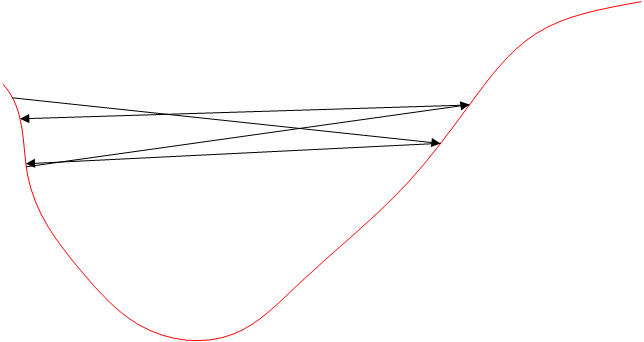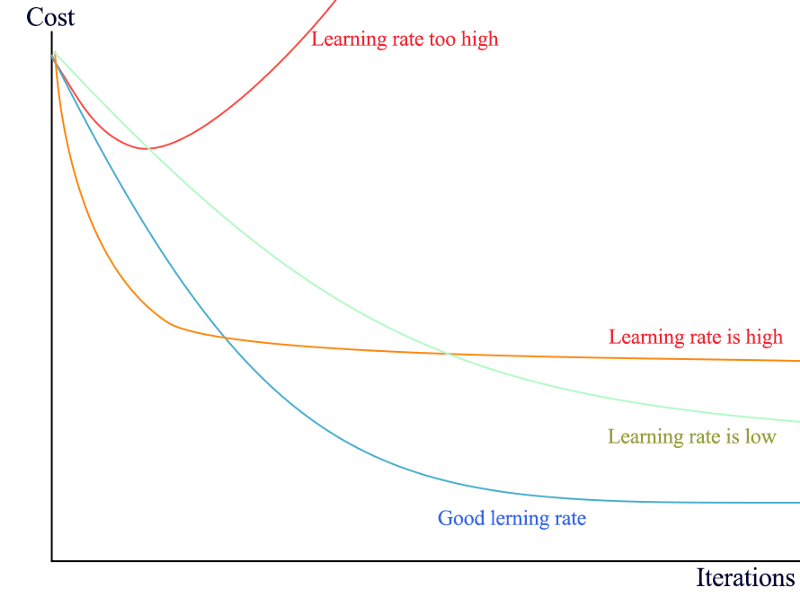# 4， 别在softmax层前面的输入施加了激活函数

softmax函数如式(4.1)所示：
S ( x i ) = exp ⁡ ( x i ) ∑ j = 1 n exp ⁡ ( x j ) (4.1) S(x_i)=\dfrac{\exp{(x_i)}}{\sum_{j=1}^n \exp(x_j)} \tag{4.1}

y = g w ( y ~ ) y ∈ R n (4.2) \mathbf{y}=g_{\mathbf{w}}(\tilde{\mathbf{y}}) \\ \mathbf{y} \in \mathbb{R}^n \tag{4.2}

y = σ ( g w ( y ~ ) ) σ ( ⋅ ) 为 激 活 函 数 y ∈ R n (4.3) \mathbf{y}=\sigma(g_{\mathbf{w}}(\tilde{\mathbf{y}}) ) \\ \sigma(\cdot) 为激活函数\\ \mathbf{y} \in \mathbb{R}^n \tag{4.3}

# 6，别忘了对你的训练数据进行打乱

e p o c h _ 1 → s h u f f l e → e p o c h _ 2 → s h u f f l e ⋯ epoch\_1 \rightarrow shuffle \rightarrow epoch\_2 \rightarrow shuffle \cdots

# 8，少用vanilla SGD优化器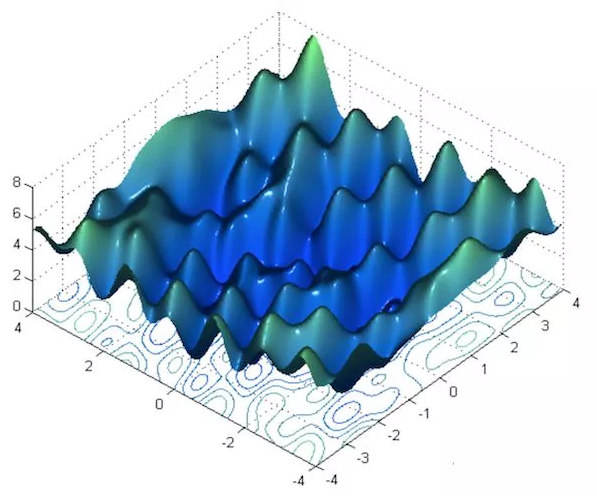# 9，检查各层梯度，对梯度爆炸进行截断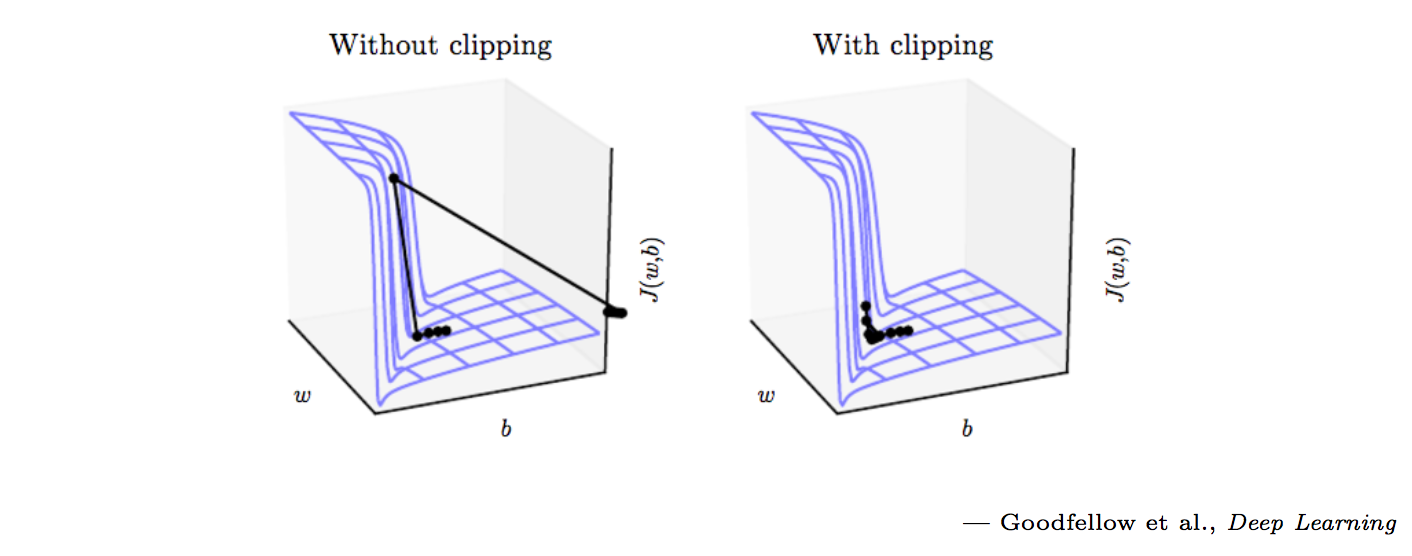tf.clip_by_value(
t,
clip_value_min, # 指定截断最小值
clip_value_max, # 指定截断最大值
name=None
)


# 11. 分类问题中的分类置信度问题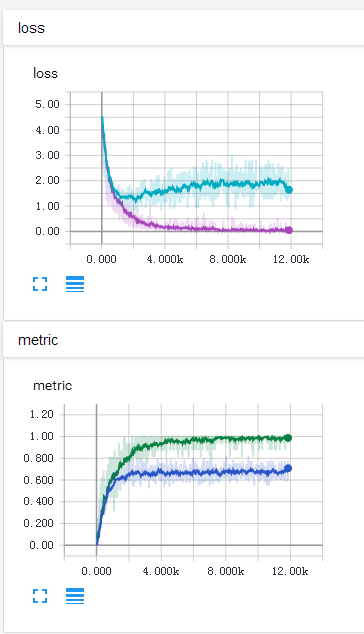[ 0.9 , 0.01 , 0.02 , 0.07 ] [0.9,0.01,0.02,0.07]

[ 0.5 , 0.4 , 0.05 , 0.05 ] [0.5,0.4,0.05,0.05]

# 12. 少在太小的批次中使用BatchNorm层

Batch Normalization，中文译作批规范化，在深度学习中是一种加快收敛速度，提高性能的一个利器，其本质和我们对输入的原数据进行0均值单位方差规范化差不多，是以batch为单位，对中间层的输出进行规范化，可以缓和内部协方差偏移(Internal Covariate Shift)的现象。其基本公式很简单，如下：
x ~ = x i − μ σ i x i n o r m = γ i ⋅ x ~ + β i (12.1) \begin{aligned} \tilde{x} &= \dfrac{x_i-\mu}{\sigma_i} \\ x_i^{\rm{norm}} &= \gamma_i \cdot \tilde{x} + \beta_i \end{aligned} \tag{12.1}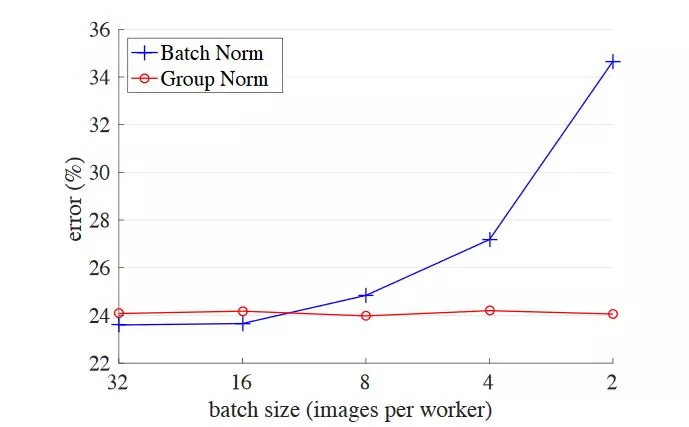# 13. 数值计算问题，出现Nan

Nan(Not An Number)是一个在数值计算中容易出现的问题，在深度学习中因为涉及到很多损失函数，有些损失函数的定义域并不是整个实数，比如常用的对数，因此一不小心就会出现Nan。在深度学习中，如果某一层出现了Nan，那么是具有传递性的，后面的层也会出现Nan，因此可以通过二分法对此进行排错。

log ⁡ ( x + ϵ ) ≈ log ⁡ ( x ) \log(x+\epsilon) \approx \log(x)

# 14. BN层放置的位置问题

BN层有两种常见的放置位置，如下图所示：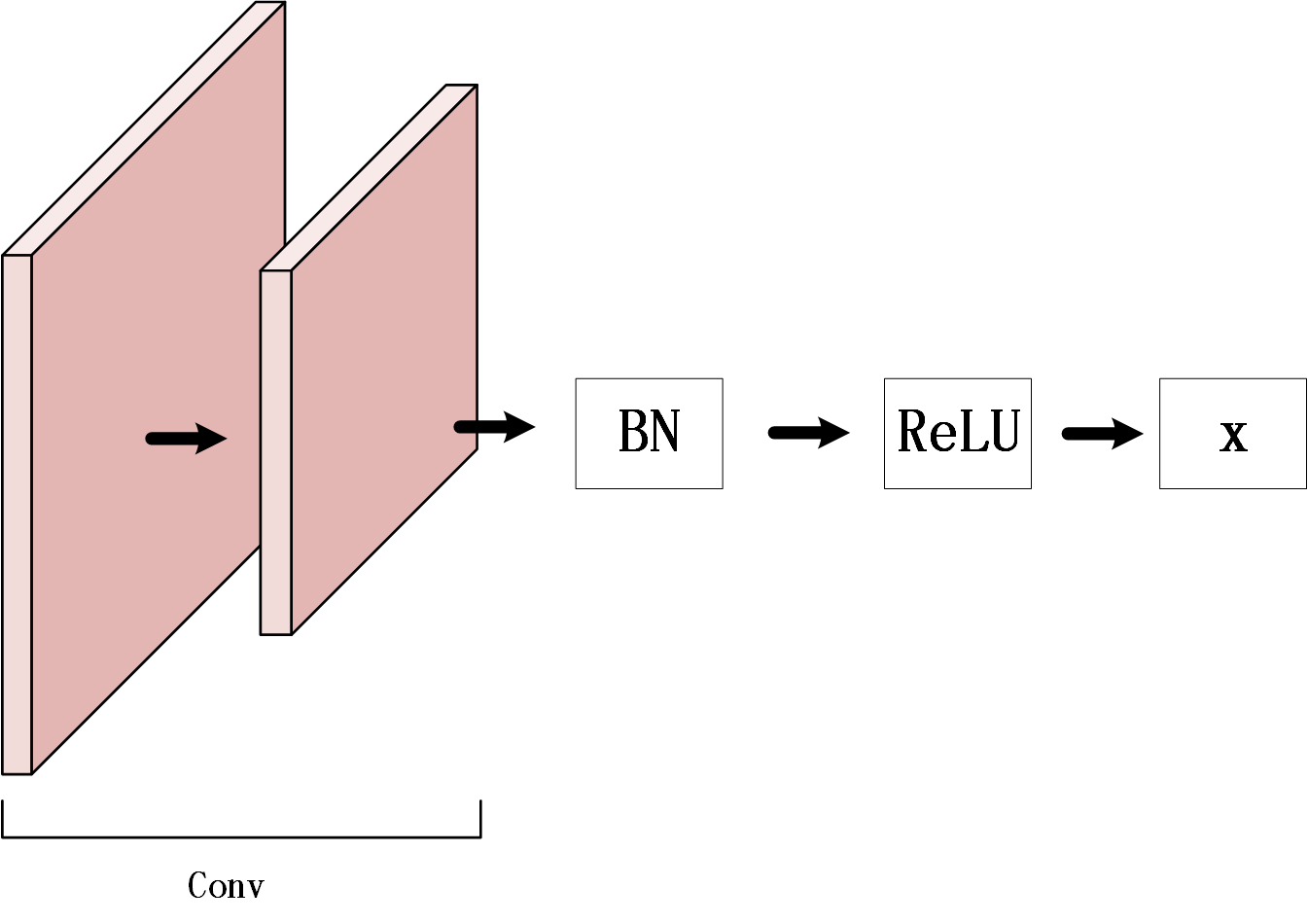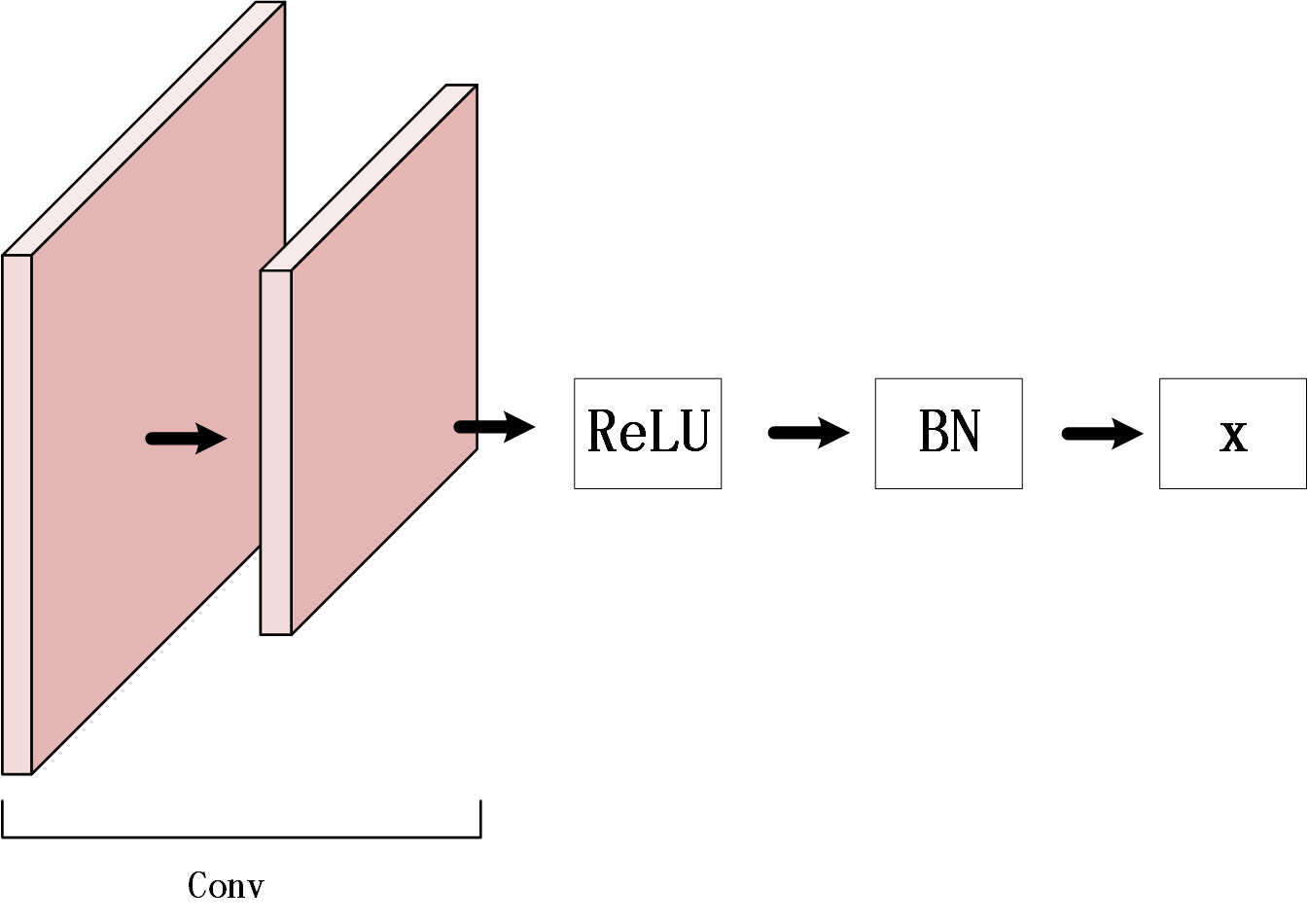Update 2020/5/18:

的作者提出的用法：

# 15. dropout层应用在卷积层中可能导致更差的性能

dropout是hinton大神与2012年提出的一种神经网络正则手段，其可以简单解释为在训练过程中，按一定概率让神经网络中的某些神经元输出为0，其原因可以有几个解释，一个是作为一种集成模型进行解释，另一个可以看成是在特征提取学习过程中给数据加入噪声，可以看成是一种数据增强的正则手段。

# 17. 初始化权值不能初始化为全0

∂ C ∂ w i , j l = a k l − 1 ∗ δ j l = a k l − 1 ∗ ∑ k ( δ k l + 1 ∗ w k , j l + 1 ∗ σ ′ ( z j l ) ) (17.1) \begin{aligned} \dfrac{\partial C}{\partial w_{i,j}^l} &= a_{k}^{l-1} * \delta_{j}^l \\ &= a_{k}^{l-1} * \sum_{k} (\delta_{k}^{l+1} * w_{k,j}^{l+1} * \sigma^{\prime}(z_{j}^l)) \end{aligned} \tag{17.1}

∂ C ∂ b j l = δ j l ∗ 1 = δ j l (17.2) \begin{aligned} \dfrac{\partial C}{\partial b_{j}^l} &= \delta_{j}^l * 1 = \delta_{j}^l \tag{17.2} \end{aligned}

# 20. KL散度出现负数

Kullback–Leibler散度，简称KL散度，也称为相对熵，是一种用于度量两个分布之间相似性的常用手段，公式如(20.1)，其中第二行形式的变形描述了相对熵的特性。
D K L ( P ∥ Q ) = ∑ i P ( i ) log ⁡ ( P ( i ) Q ( i ) ) = ∑ i { P ( i ) log ⁡ ( P ( i ) ) − P ( i ) log ⁡ ( Q ( i ) ) } (20.1) \begin{aligned} \mathrm{D}_{\mathrm{KL}}(P \Vert Q) &= \sum_{i} P(i) \log(\dfrac{P(i)}{Q(i)}) \\ &= \sum_{i}\{ P(i)\log(P(i)) - P(i)\log(Q(i)) \} \end{aligned} \tag{20.1}

# Reference

. Janocha K, Czarnecki W M. On loss functions for deep neural networks in classification[J]. arXiv preprint arXiv:1702.05659, 2017.(Overview about loss function used in DNN)
. tf.one_hot()进行独热编码
. 曲线拟合问题与L2正则
. Kinga D, Adam J B. A method for stochastic optimization[C]//International Conference on Learning Representations (ICLR). 2015, 5.
. <深度学习系列>深度学习中激活函数的选择
. 机器学习之特征归一化（normalization）
. 机器学习模型的容量，过拟合与欠拟合
. 在机器学习中epoch, iteration, batch_size的区别
. tf.clip_by_value
. 梯度截断的tensorflow实现
. 均方误差(MSE)和均方根误差(RMSE)和平均绝对误差(MAE)
. Du S S, Zhai X, Poczos B, et al. Gradient Descent Provably Optimizes Over-parameterized Neural Networks[J]. arXiv preprint arXiv:1810.02054, 2018.
. The inconsistency between the loss curve and metric curve?
. Ioffe S, Szegedy C. Batch normalization: accelerating deep network training by reducing internal covariate shift[C]// International Conference on International Conference on Machine Learning. JMLR.org, 2015:448-456.
. Wu Y, He K. Group normalization[J]. arXiv preprint arXiv:1803.08494, 2018.
. Park S, Kwak N. Analysis on the dropout effect in convolutional neural networks[C]//Asian Conference on Computer Vision. Springer, Cham, 2016: 189-204.
. Where should I place dropout layers in a neural network?
. Hinton G E, Srivastava N, Krizhevsky A, et al. Improving neural networks by preventing co-adaptation of feature detectors[J]. arXiv preprint arXiv:1207.0580, 2012.
. Why do I never see dropout applied in convolutional layers?
. L1正则
. 《深度学习系列》反向传播算法的公式推导
. 训练集，测试集，检验集的区别与交叉检验
. Kullback–Leibler divergence
. Probability space
. 如何理解K-L散度（相对熵）
. KL Divergence produces negative values
. Chen G, Chen P, Shi Y, et al. Rethinking the Usage of Batch Normalization and Dropout in the Training of Deep Neural Networks[J]. arXiv preprint arXiv:1905.05928, 2019.
. https://forums.fast.ai/t/rethinking-batchnorm-dropout-combine-together-for-independent-component-layer/46232

11-26
07-181万+02-15377
11-18252
07-26530
06-15122
08-1083
01-30812
07-14207
10-251万+